### Home > CALC > Chapter 6 > Lesson 6.1.1 > Problem6-9

6-9.
1. Find the inverse, f −1 (x), for the following functions. Homework Help ✎

1. f(x) = 2x

2. f(x) = log3 x

3. f(x) = 1oge x

4. f(x) = 5 · 9 x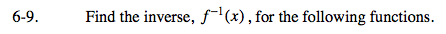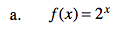Solve for x: log2y = x
Then 'switch' the input and the output.

f −1(x) = log2x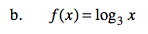This is the reverse of part (a).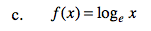Recall that e is a constant. (It's value is between 2 and 3)

f −1(x) = ex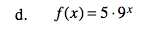Be sure to divide both sides by 5 before you solve for x .